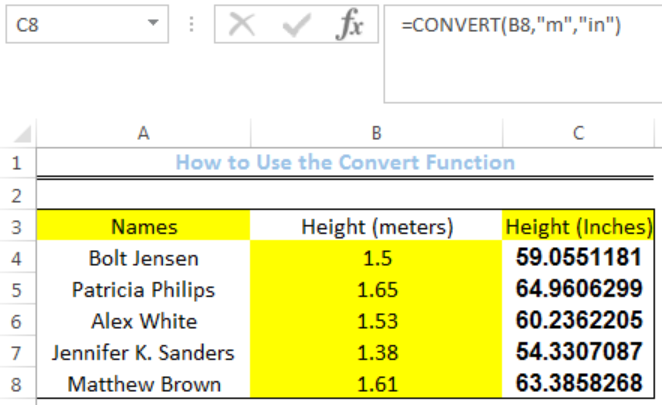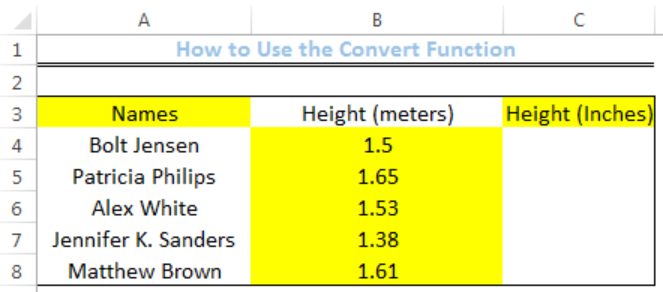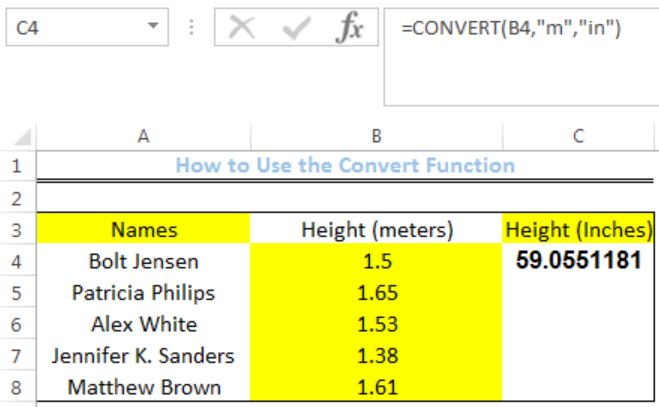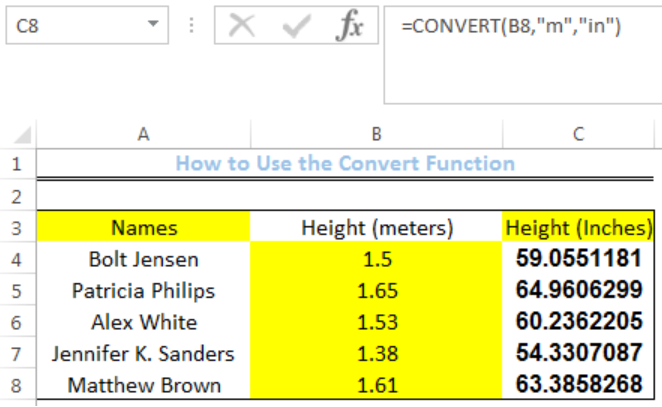Get instant live expert help with Excel or Google Sheets“My Excelchat expert helped me in less than 20 minutes, saving me what would have been 5 hours of work!”

#### Post your problem and you’ll get Expert help in seconds.

Your message must be at least 40 characters
Our professional Expert are available now. Your privacy is guaranteed.

# Excel CONVERT Function

We can use the excel CONVERT FUNCTION to convert a number from one measurement to another. Some measurements are Meters, Kilometers, Fahrenheit, Celsius, Inches, Gram, etc. The steps below will walk through the process.Figure 1: How to Use the Convert Function

## Syntax

`=CONVERT(number, from_unit, to_unit)`

• Where “Number” is the number to convert or the cell containing the number
• “From unit” is the current unit of the number
• “To unit” is the output unit that is required

## Formula

`=CONVERT(B4,"m","in")`

## Setting up the Data

• We will set up the data by inputting the Names of some students into Column A
• Column B will contain the HEIGHT of the students in meters
• Column C is where we want the formula to return the result for the HEIGHT of the students in inchesFigure 2: Setting up the Data

## Convert From Meters to Inches

• We will click on Cell C4
• We will insert the formula below into the cell
`=CONVERT(B4,"m","in")`
• We will press the enter keyFigure 3: Conversion from Meters to Inches of Cell B4

• We will click on Cell C4 again
• We will double-click on the fill handle (the small plus sign at the bottom right of Cell C4) and drag down to copy the formula into the other cellsFigure 4: Conversion from Meters to Inches of Cells of Column B

## Explanation

`=CONVERT(B4,"m","in")`

The value in Cell B4 is reads as meters and the convert function returns the inches equivalent of the number.

## Instant Connection to an Expert through our Excelchat Service

Most of the time, the problem you will need to solve will be more complex than a simple application of a formula or function. If you want to save hours of research and frustration, try our live Excelchat service! Our Excel Experts are available 24/7 to answer any Excel question you may have. We guarantee a connection within 30 seconds and a customized solution within 20 minutes.

### Did this post not answer your question? Get a solution from connecting with the expert.Another blog reader asked this question today on Excelchat:## Subscribe to Excelchat.coAnother blog reader asked this question today on Excelchat: